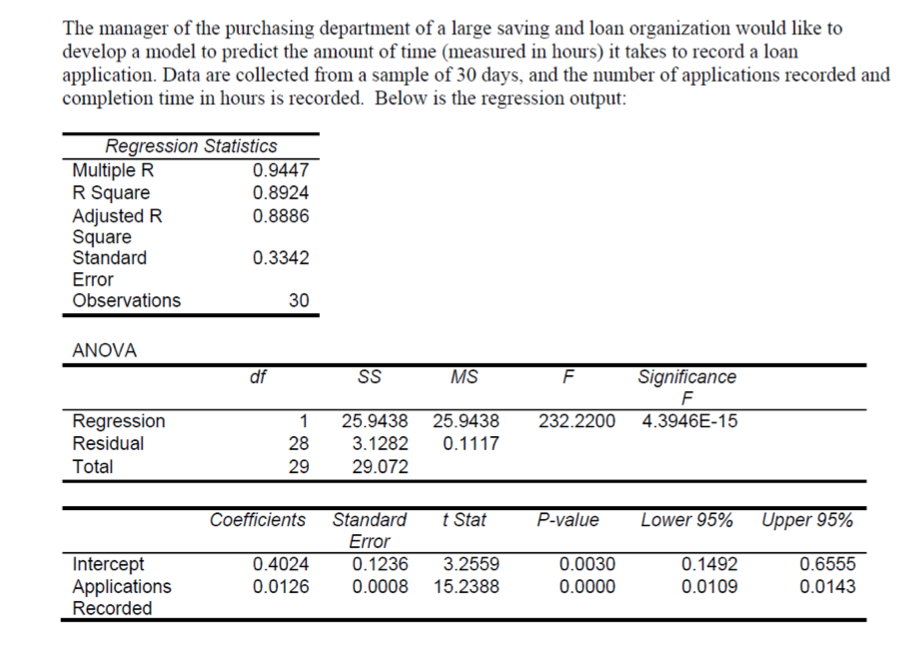# The manager of the purchasing department of a large saving and loan organization would like todevelop a model to predict the amount of time (measured in hours) it takes to record a loanapplication. Data are collected from a sample of 30 days, and the number of applications recorded andcompletion time in hours is recorded. Below is the regression output:Regression StatisticsMultiple RR SquareAdjusted RSquareStandard0.94470.89240.88860.3342ErrorObservations30ANOVAMSSignificancedfSS4.3946E-15Regression25.9438 25.9438232.2200Residual283.12820.1117Total2929.072CoefficientsStandardP-valueLower 95%t StatUpper 95%Error0.1492InterceptApplicationsRecorded0.40240.12363.25590.00300.65550.01430.01260.000815.23880.00000.0109

Question
1 views

Referring to the above scenario, interpret the coefficient attached to variable applications recorded:

Referring to the above scenario, interpret R Square:

Referring to the above scenario, interpret the F-statistic in the ANOVA analysis:

Referring to above scenario, predict the amount of time it would take on average to process 150 invoices:help_outlineImage TranscriptioncloseThe manager of the purchasing department of a large saving and loan organization would like to develop a model to predict the amount of time (measured in hours) it takes to record a loan application. Data are collected from a sample of 30 days, and the number of applications recorded and completion time in hours is recorded. Below is the regression output: Regression Statistics Multiple R R Square Adjusted R Square Standard 0.9447 0.8924 0.8886 0.3342 Error Observations 30 ANOVA MS Significance df SS 4.3946E-15 Regression 25.9438 25.9438 232.2200 Residual 28 3.1282 0.1117 Total 29 29.072 Coefficients Standard P-value Lower 95% t Stat Upper 95% Error 0.1492 Intercept Applications Recorded 0.4024 0.1236 3.2559 0.0030 0.6555 0.0143 0.0126 0.0008 15.2388 0.0000 0.0109 fullscreen
check_circle

Step 1

From the regression output, the coefficient of application recorded is 0.0126.

Interpretation:

The slope 0.0126 indicates that for each additional application recorded the mean amount of time will increase by 0.0126 hours.

Step 2

R-square:

The value of R-square is 0.8924.

There is 89.24% of variability in the amount of time can be explained by the number of applications recorded.

Step 3

From the regression output, the F test statistic is 232.2200 and the p-value is 0.0000.

Consider the significance level is 0.05. Here, the p-value is too small compared to s...

### Want to see the full answer?

See Solution

#### Want to see this answer and more?

Solutions are written by subject experts who are available 24/7. Questions are typically answered within 1 hour.*

See Solution
*Response times may vary by subject and question.
Tagged in

### Other